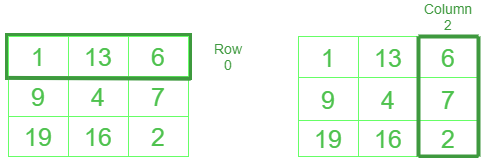# How to access a NumPy array by column

Accessing a NumPy based array by specific Column index can be achieved by the indexing. Let’s discuss this in detail.

NumPy follows standard 0 based indexing.Row and column in NumPy are similar to Python List

Examples:

```Given array : 1 13 6
9  4 7
19 16 2

Input: print(NumPy_array_name[ :,2])

# printing 2nd column
Output: [6 7 2]

Input: x =  NumPy_array_name[ :,1]
print(x)

# storing 1st column into variable x
Output:  [13 4 16]
```

Method #1: Selection using slices

Syntax :

For column : numpy_Array_name[  : column,]

For row : numpy_Array_name[ row,:  ]

## Python3

 `# Python code to select row and column ` `# in NumPy ` ` `  `import` `numpy as np ` ` `  `array ``=` `[[``1``, ``13``, ``6``], [``9``, ``4``, ``7``], [``19``, ``16``, ``2``]] ` ` `  `# defining array ` `arr ``=` `np.array(array) ` ` `  `print``(``'printing array as it is'``) ` `print``(arr) ` ` `  `print``(``'printing 0th row'``) ` `print``(arr[``0``, :]) ` ` `  `print``(``'printing 2nd column'``) ` `print``(arr[:, ``2``]) ` ` `  `# multiple columns or rows can be selected as well ` `print``(``'selecting 0th and 1st row simultaneously'``) ` `print``(arr[:,[``0``,``1``]])`

Output :

```printing array as it is
[[ 1 13  6]
[ 9  4  7]
[19 16  2]]
printing 0th row
[ 1 13  6]
printing 2nd column
[6 7 2]
selecting 0th and 1st row simultaneously
[[ 1 13]
[ 9  4]
[19 16]]
```

Method #2: Using Ellipsis

Syntax :

For column : numpy_Array_name[,column]

For row : numpy_Array_name[row,]

where ‘‘ represents no of elements in the given row or column

Note: This is not a very practical method but one must know as much as they can.

## Python3

 `# program to select row and column ` `# in numpy using ellipsis ` ` `  `import` `numpy as np ` ` `  `# defining array ` `array ``=` `[[``1``, ``13``, ``6``], [``9``, ``4``, ``7``], [``19``, ``16``, ``2``]] ` ` `  `# converting to numpy array ` `arr ``=` `np.array(array) ` ` `  `print``(``'printing array as it is'``) ` `print``(arr) ` ` `  `print``(``'selecting 0th column'``) ` `print``(arr[..., ``0``]) ` ` `  `print``(``'selecting 1st row'``) ` `print``(arr[``1``, ...]) `

Output :

```printing array as it is
[[ 1 13  6]
[ 9  4  7]
[19 16  2]]
selecting 0th column
[ 1  9 19]
selecting 1st row
[9 4 7]
```

Attention geek! Strengthen your foundations with the Python Programming Foundation Course and learn the basics.

To begin with, your interview preparations Enhance your Data Structures concepts with the Python DS Course.

My Personal Notes arrow_drop_upCheck out this Author's contributed articles.

If you like GeeksforGeeks and would like to contribute, you can also write an article using contribute.geeksforgeeks.org or mail your article to contribute@geeksforgeeks.org. See your article appearing on the GeeksforGeeks main page and help other Geeks.

Please Improve this article if you find anything incorrect by clicking on the "Improve Article" button below.

Article Tags :

1

Please write to us at contribute@geeksforgeeks.org to report any issue with the above content.